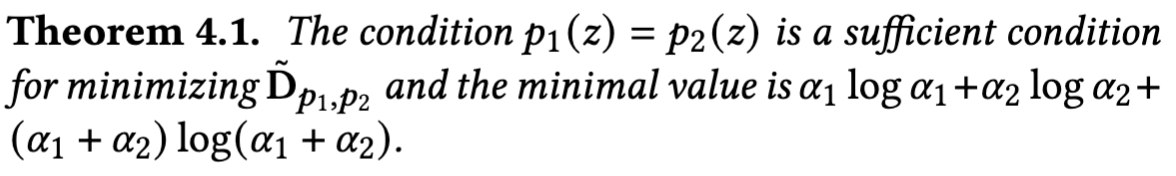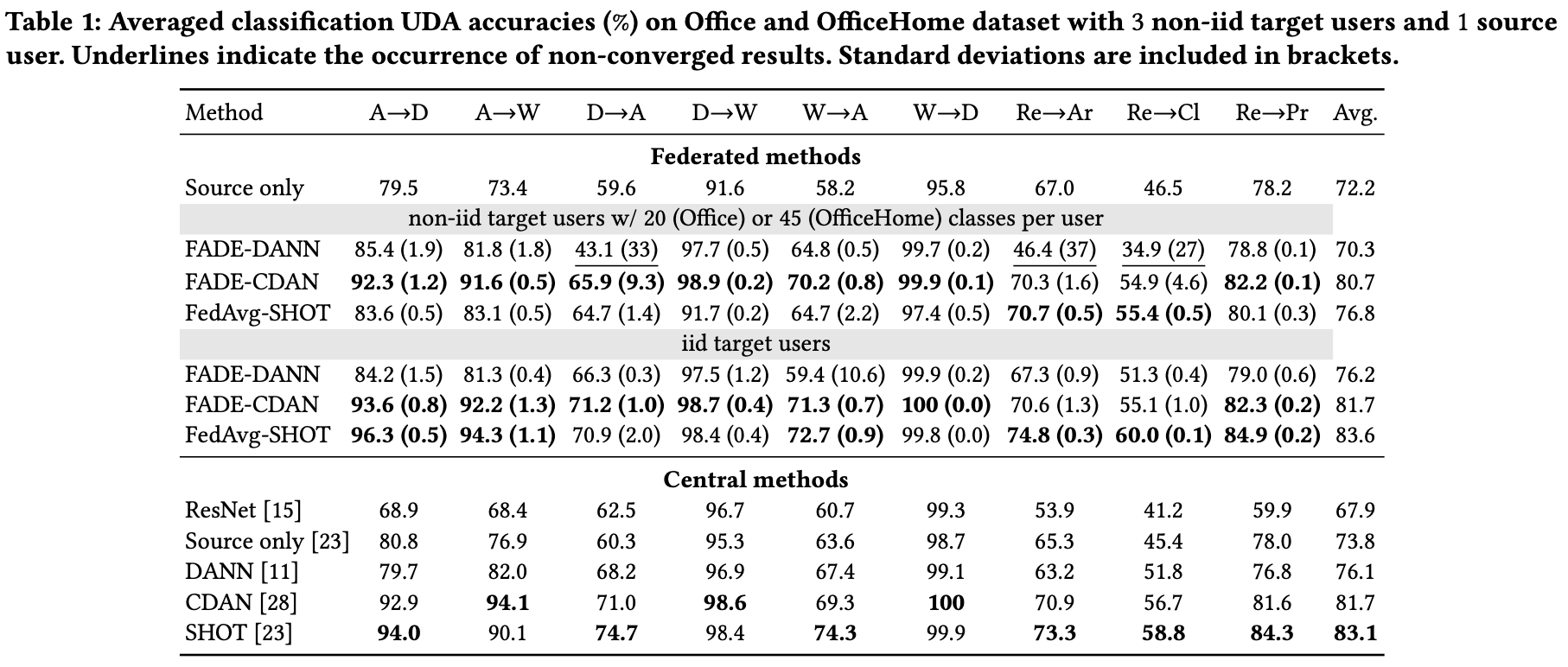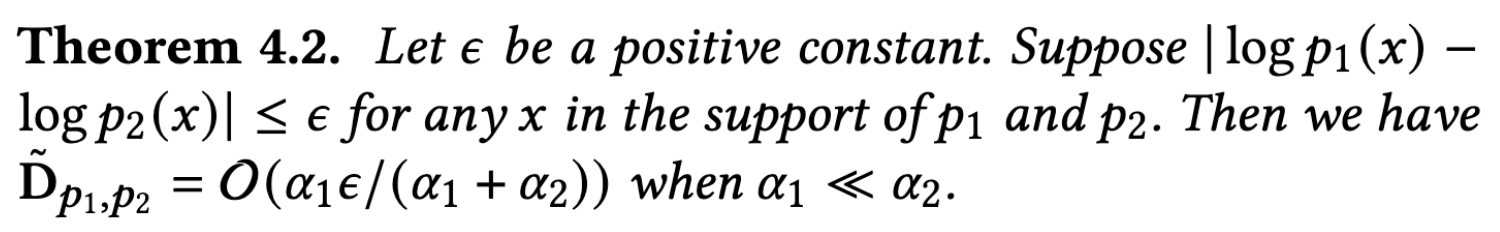# Federated Adversarial Debiasing for Fair and Trasnferable Representations

### Abstract

Federated learning is a distributed learning framework that is communication efficient and provides protection over participating users' raw training data. One outstanding challenge of federate learning comes from the users' heterogeneity, and learning from such data may yield biased and unfair models for minority groups. While adversarial learning is commonly used in centralized learning for mitigating bias, there are significant barriers when extending it to the federated framework. In this work, we study these barriers and address them by proposing a novel approach Federated Adversarial DEbiasing (FADE). FADE does not require users' sensitive group information for debiasing and offers users the freedom to opt-out from the adversarial component when privacy or computational costs become a concern. We show that ideally, FADE can attain the same global optimality as the one by the centralized algorithm. We then analyze when its convergence may fail in practice and propose a simple yet effective method to address the problem. Finally, we demonstrate the effectiveness of the proposed framework through extensive empirical studies, including the problem settings of unsupervised domain adaptation and fair learning.

Type
Publication
In The 27th ACM SIGKDD International Conference on Knowledge Discovery & Data Mining

The distribution shift between two groups can be debiased in the representation space. For example, when the encoder $G$ is fixed, a discriminator network $D$ can be trained to criticize the group discrepancy of samples from two groups 1. Meanwhile, we debias the representations by training the encoder $G$ to maximize the discrimination error when fixing the discriminator. For central learning, the objective is $$\min_f \max_g \mathbb{E}_{(x,y, g)} [ \ell_c(f,G; x,y) + \ell_d (D,G; x,g) ]$$ where the debiasing loss is $$\ell_d = \mathbb{I}(g=0) \log(D(G(x))) + \mathbb{I}(g=1) \log(1 - D(G(x)))$$ and the classifier loss is $$\ell_c = \text{XEnt}(f(G(x)), y)$$ where $\text{XEnt}$ is the cross-entropy loss.

However, such a debiasing method is not feasible in a federated setting where users' data will not be aggregated due to the privacy concern. A recent work2 propose to do the adversarial debiasing on the gathered representations. Either the source domain users or the target domain users has to send their data presentations to the other group. First, this will increase the communication burden among users. When $M$ source domain users and $N$ target domain users are involved, the communication occurs $MN$ times. Second, sharing representations is not safe for privacy, as it is easy to reverse-engineering the representations to obtain the input samples. Especially, when the encoder is shallow.

Instead, our method, Federated Adversarial DEbiasing (FADE), does not require users to share their data but only sharing an additional discriminator sub-network. Just like FedAvg3, the shared model help to transfer the useful knowledge in the data while keeping raw data locally.

However, such a method raises new challenges. First, we will find that the $\ell_d$ has only one side objective. For example, for group $g=1$, the loss of group $0$ will be missing. Formally, we will write the federated objective as $$\min_{f,G} \mathcal{L}(f, G) = \sum_{g=1}^E \sum_{i=1}^{m_g} L_{i,g}(f, G),$$ $$L_{i,g} (f, G) = L_i^{task}(f, G) + \lambda \max_D L_{i,g}^{adv} (G, D),$$ where $L_i^{task}(f, G)$ is the classification loss for the $i$-th user, $L_{i,g}^{adv} (G, D)$ is the adversarial loss and $m_g$ is the number of users in group $g$. For the two-group case, the adversarial loss can be \begin{aligned} L_{i,g}^{adv} (G, D) = \mathbb{E}_{x\sim p_i(x)} \left[ \mathbb{I}(g=0) \log(D(G(x))) \right. \\ %\mu + \tau\times\eta = \theta \sim N(\mu , \tau^2) \left. +\mathbb{I}(g=1) \log(1 - D(G(x))) \right]. %+ \mathbb{I}(g=1) \log (1 - D(G(x))) \end{aligned} The critical problem is if the optimization can converge when the counterpart group is missing. In other words, we want ask if the distribution matching is a sufficient condition for the minimization.As shown in Theorem 1.4, it is a sufficient condition for the minimizing the model-measured discrepancy $\tilde D$ between $p_1$ and $p_2$. We also demonstrate the effectiveness by experiments on unsupervised domain adaptation (UDA) benchmarks. The FADE-based achieve performance comparable to central versions. In non-iid and autonomous-user-involving (2 users per round), FADE outperforms the baselines.### Impact of Imbalanced Groups

We also notice a possible negative impact due to the imbalance of group users. Suppose the ratio of two group users are $\alpha_1$ and $\alpha_2$, respectively. Then the sensed discrepancy will be biased as the imbalance is more severer.To fix this, we propose re-weight the losses according to the loss scales. That is $\hat \ell = - \ell^2 / 2$ which was used for fair-federated learning. We compare the vanilla loss versus the squared loss in Fig 4. As more target users are involved, the imbalance is worsened and the squared loss could improve the drop of vanilla losses.

We also conduct imbalanced experiments in fair federated learning. Squared loss is preferred as imbalance data present, while vanilla loss is preferred in reversed cases.

### Impact of Non-iid Users

In addition, the adversarial training may not only debias unwanted distribution shift but also important discriminative information, as class-wise non-iid distributions are present in federated users. The unwanted debiasing is named user collapse in the scope of this paper. We argue that using a regularization to limit the user collapse is plausible. For example, a regularization conditioned on the possible classes is helpful4.

1. Ganin, Y., & Lempitsky, V. (2015). Unsupervised Domain Adaptation by Backpropagation. ICML, 1180–1189. http://proceedings.mlr.press/v37/ganin15.html ↩︎

2. Peng, X., Huang, Z., Zhu, Y., & Saenko, K. (2019, September 25). Federated Adversarial Domain Adaptation. ICLR. https://openreview.net/forum?id=HJezF3VYPB ↩︎

3. McMahan, B., Moore, E., Ramage, D., Hampson, S., & Arcas, B. A. y. (2017). Communication-Efficient Learning of Deep Networks from Decentralized Data. AISTAT, 1273–1282. http://proceedings.mlr.press/v54/mcmahan17a.html ↩︎

4. Long, M., Cao, Z., Wang, J., & Jordan, M. I. (2018). Conditional Adversarial Domain Adaptation. ArXiv:1705.10667 [Cs]. http://arxiv.org/abs/1705.10667 ↩︎##### Junyuan Hong
###### Postdoctoral Fellow

My research interests include data privacy and trustworthy machine learning.